# Design, Modeling and Optimization of Hybrid Photovoltaic/Wind Turbine System with Battery Storage: Application to Water Pumping

Design, Modeling and Optimization of Hybrid Photovoltaic/Wind Turbine System with Battery Storage: Application to Water Pumping

Katia Tadjine Djamila RekiouaSaloua Belaid Toufik Rekioua Pierre-Olivier Logerais

Laboratoire de Technologie Industrielle et de l'Information (LTII), Faculté de Technologie, Université de Bejaia, Bejaia 06000, Algeria

Université Paris Est Créteil, CERTES, IUT de Sénart-Fontainebleau, 36 rue Georges Charpak, Lieusaint 77567, France

Corresponding Author Email:
djamila.ziani@univ-bejaia.dz
Page:
655-667
|
DOI:
https://doi.org/10.18280/mmep.090312
1 May 2022
|
Accepted:
22 June 2022
|
Published:
30 June 2022
| Citation

OPEN ACCESS

Abstract:

This paper addresses the modeling, design and optimization of a photovoltaic/wind turbine/batteries system. Tests are made within the area of Bejaia (Algeria), where the solar and wind energies are very exploitable due to its geographical location. The design of the studied system uses the total incident energy approach to determine the size of the photovoltaic and wind generators. The different sources are connected by converters to the DC bus, which optimizes the operation of maximization whatever the weather conditions. The strategy optimization of the PV system is made by three MPPT methods (Pertub & Observ (P&O), Incremental & conductance (INCcond) and fuzzy logic controller (FLC). As for the wind turbine, three MPPT methods have been investigated (Optimal torque control (OTC), Gradient Method (GM) and Fuzzy logic controller (FLC). Following the comparisons of the power, efficiency and response time, the method presenting the best performances is incorporated in the studied system. The simulation is built using Matlab/Simulink. The results obtained during four days of each season (summer, autumn, winter, and spring) are shown and discussed to demonstrate the applicability of the suggested system. To control the water pumping hybrid system, a power control is proposed.

Keywords:

modeling, optimization, photovoltaic panels, wind turbine, MPPT, design

1. Introduction

Intermittent nature of wind and photovoltaic energy production complicates the continuous operating of an electrical renewable system. By storing or restituting the surplus energy when needed, an energy storage facility is necessary to balance the demand and the supply of power. As a result, hybrid systems being both environmentally friendly and safer, are designed [1, 2]. To obtain the maximum power point of solar panels [3-10] and wind turbines [11-17], a variety of MPPTs are used. They all aim to maximize the power, but differ from each other in their performances.

In this work, the modeling, design and optimization of a hybrid photovoltaic/wind turbine water pumping system with batteries is presented. Batteries are inserted to ensure the pump demand during the periods when there is neither solar irradiance nor high wind speeds. Measurements of the wind speed, temperature and solar radiation profiles were performed during four days belonging to different seasons (winter, spring, summer and autumn) using a data acquisition system at the laboratory. An application is made for water pumping during the different seasons in a coastal Mediterranean region. The proposed sizing method involves the annual monthly average of the total incident energy . This approach allows us to determine the size of the two generators. The different parameters of PV, wind turbine and batteries are identified at the laboratory to use them in simulation and to obtain more realistic models. The strategy optimization for the PV system is made by three MPPT methods (Pertub. & Observ (P&O) [3-5], Incremental & conductance (IncCond) [6-8] and fuzzy logic controller (FLC) [9, 10]. And, for the wind turbine, three MPPT methods have been investigated (Optimal torque control (OTC) [11, 12], Gradient Method (GM) [13, 14] and Fuzzy logic controller (FLC) [15-17]. Subsequently to comparisons, the proposed optimization strategy is based on the FLC MPPT method for the two generators, owing to its better performances in terms of power, efficiency and response time. The results clearly demonstrate the practicality of the suggested method on various days corresponding to the different seasons (winter, spring, summer and autumn). The proposed supervision for the water pumping system is used to satisfy the level of the pump needs and to protect the batteries used.

2. System Description

In our study, a renewable energy system based on batteries has been chosen (Figure 1). Its different components are connected to static converters and to a management supervisor to follow the various powers.

3. Design of Hybrid (PV/Wind Turbine/Batteries Storage) System

The sizing of a system is an essential step in determining its optimal design. The method using the annual monthly average of the total incident energy is considered in our work.

## 1.png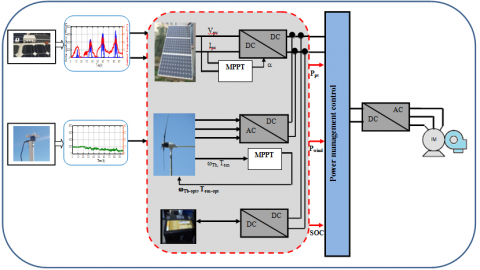Figure 1. System description

The monthly energy produced is denoted by Epv,m for the PV generator and Ewind,m for the wind generator (m=1, ..., 12 are the number of the months during a year).

The area of the generators necessary to achieve full load coverage during the worst month is calculated as follows:

$S_{p v}=\max \left(\frac{E_{\text {Load }, m}}{E_{p v, m}}\right)$

$S_{\text {wind }}=\max \left(\frac{E_{\text {Load }, m}}{E_{\text {wind }, m}}\right)$       (1)

where,

$E_{p v}=\eta_{p v} \cdot S_{p v} \cdot E_{i r r}$

$E_{w i n d}=P_{w i n d}=(1 / 2) \cdot \rho \cdot S \cdot V_{w i n d}^{3} \cdot C_{p} \cdot \Delta t$

$E_{\text {Load }}=S_{p v} \cdot E_{p v}+S_{\text {wind }} \cdot E_{\text {wind }}$            (2)

with, Spv: the PV total area, ηpv: the PV efficiency, Eirr: the solar irradiance on a tilted plane.

The monthly energy produced by the system per unit of area is denoted Epv,m for the photovoltaic energy and Ewind,m for the wind energy and EL,m represents the energy required by the load (where m=1, 2, ..., 12 are the months of the year). We have:

\begin{aligned} E_{p v, m} &=\left(\sum_{m=1}^{12} E_{p v}\right) / 12 \\ E_{\text {wind }, m} &=\left(\sum_{m=1}^{12} E_{\text {wind }}\right) / 12 \\ E_{\text {Load }, m} &=\left(\sum_{m=1}^{12} E_{\text {Load }}\right) / 12 \end{aligned}          (3)

The limit value f=1 implies that the load is totally supplied by the PV power, whereas f=0 means that the load is entirely supplied by the wind power.

Therefore, the areas of the two PV and wind generators are given respectively by:

$S_{p v}=f\left(E_{\text {Load,ave }} / E_{p v, a v e}\right) \\ S_{\text {wind }}=(1-f)\left(E_{\text {Load,ave }} / E_{\text {wind,ave }}\right)$                   (4)

The number of PV panels and wind turbine are determined by the following equations:

$S_{p v, \text { final }}=N_{p v} \cdot S_{p v, u n i t} \\ S_{\text {wind,final }}=N_{\text {wind }} \cdot S_{\text {wind,unit }}$        (5)

The average consumed energy is given by:

$E_{\text {load-ave }}=E_{p v, a v e} \cdot S_{p v, u n i t}+E_{\text {wind,ave }} \cdot S_{\text {wind }, \text { unit }}$        (6)

The different calculations are summarized in Table 1. It illustrates the possible configurations of the coupling of the photovoltaic system with the wind power system. After calculations, the different energies are found in Table 1: Epv,m=31.59 kWh/m², Ewind,m=54.86 kWh/m² and ELoad,m=231.09 kWh.

Besides, in Table 2, it is noticeable that the only value that is closer to the load value (231.09 kWh) is 244.40 kWh, hence the optimal configuration corresponds to f=0.9 (six PV panels +one wind turbine). The calculation of the battery capacity gives two batteries of (100 Ah, 12V).

Table 1. Different possible configurations of PV and wind systems

 Eir(kWh/m2) Vwind(m/s) Epv(kWh/m2) Ewind(kWh/m2) ELoad(kWh) 160.00 3.06 28.08 21.77 111.92 162.00 3.06 28.43 20.36 107.52 186.00 4.72 32.64 79.89 319.16 185.00 4.17 32.47 53.31 226.88 195.00 4.17 34.22 55.09 235.32 190.00 3.89 33.35 43,28 193.27 200.00 4.44 35.10 66.50 275.98 202.00 4.44 35.45 66.50 276.43 193.00 4.72 33.87 77.31 311.83 180.00 3.89 31.59 44.72 195.99 157.00 3.61 27.55 34.59 155.64 150.00 5.00 26.33 94.96 363.16 4.10 31.59 54.86 231.09

Table 2. Calculation of the number of panels and wind turbine

 f Spv (m2) Swind (m2) Npv Nwind Spvfinal (m2) Swind,final (m2) ELmean (kWh) 0 0.00 4.21 0 2 0.00 6.93 109.71 0.1 0.73 3.79 1 2 1.30 6.93 141.30 0.2 1.46 3.37 2 1 2.60 3.46 118.04 0.3 2.19 2.95 2 1 2.60 3.46 118.04 0.4 2.93 2.53 3 1 3.90 3.46 149.63 0.5 3.66 2.11 3 1 3.90 3.46 149.63 0.6 4.39 1.69 4 1 5.20 3.46 181.22 0.7 5.12 1.26 4 1 5.20 3.46 181.22 0.8 5.85 0.84 5 1 6.50 3.46 212.81 0.9 6.58 0.42 6 1 7.80 3.46 244.40 1.0 7.32 0.00 6 0 7.80 0.00 189.54
4. Proposed System Modeling

4.1 Photovoltaic panels model

The electrical characteristic is obtained by :

$I_{p v}=I_{p h}-I_{d}-I_{R s h}$

$I_{p v}=I_{p h}-I_{0}\left[\exp \left(\frac{q\left(V_{p v}+I_{p v} \cdot R_{s}\right)}{A N_{s} k T_{j}}\right)-I\right]-\frac{V_{p v}+R_{s} \cdot I_{p v}}{R_{s h}}$           (7)

The photocurrent Iph, the polarization current Id and the shunt current are respectively given as [18, 19]:

$I_{p h}=P_{1} \cdot E_{s} \cdot\left[1+P_{2} \cdot\left(E_{s}-E_{s-S T C}\right)+P_{3} \cdot\left(T_{j}-T_{j-S T C}\right)\right]$

$I_{d}=P_{4} \cdot T_{j}^{3} \cdot \exp \left(-\frac{E_{g}}{K \cdot T_{j}}\right) \cdot\left[\exp \left(\frac{q \cdot\left(V_{p v}+R_{s} \cdot I_{p v}\right)}{A \cdot n_{s} \cdot k \cdot T_{j}}\right)-1\right]$

$I_{s h}=\frac{\left(V_{p v}+R_{s} \cdot I_{p v}\right)}{R_{s h}}$                (8)

where, Es is the solar irradiance on the panel plane; Es-STC the solar irradiance at STC conditions, Tj-STC the STC panel temperature and P1, P2, P3 and P4 are constant parameters .

So, Eq. (7) can be written as:

$I_{p v}=P_{1} \cdot E_{s} \cdot\left[\begin{array}{l}1+P_{2} \cdot\left(E_{s}-E s_{s T C}\right) \\ +P_{3} \cdot\left(T_{j}-T_{S T C}\right)\end{array}\right]-P_{4} \cdot T_{j}^{3} \cdot \exp \left(-\frac{E_{g}}{K \cdot T_{j}}\right)$

$x\left[\exp \left(q \cdot \frac{\left(V_{p v}+R_{s} \cdot I_{p v}\right)}{A \cdot n_{s} \cdot K \cdot T_{j}}\right)-1\right]-\frac{\left(V_{p v}+R_{s} \cdot I_{p v}\right)}{R_{s h}}$               (9)

Eq. (9) is solved with a numerical resolution (Figure 2) and by the use of PV data sheets (Appendix):

$\left\{\begin{array}{c}I\left(V_{o c}\right)=0 \\ I(0)=I_{s c} \\ I\left(V_{m p p}\right)=I_{m p p} \\ \left.\frac{d P}{d V}\right|_{P=P_{m p p}}=I_{m p p}+\left.\frac{d I}{d V}\right|_{V=V_{m p p}} ^{I=I_{m p p}}=0\end{array}\right.$                  (10)

Three extra equations are necessary to obtain all the seven parameters. The parameter P2 must be calculated using sites with varied solar irradiance values. Points with various temperatures are also required for the parameter P3. A convergence test is made on the function F(X, X0), where X0 and X are respectively the initial conditions and the calculated parameters .

## 1.png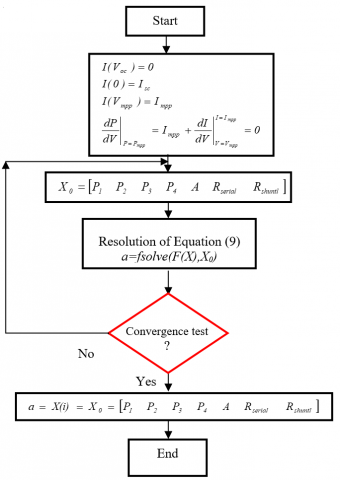Figure 2. Flowchart for the resolution of the seven parameters

A method based on optimization techniques has been developed to find the extrema for the function indicated. Its principle is simple; it consists firstly of defining an objective function, called a criterion. This criterion e depends on a set of parameters grouped in a vector p. The objective function refers to the error between the practical results and those of the simulation. It is given by:

$e(p)=\frac{{{I}_{pv-\exp }}-{{I}_{pv-sim}}}{{{I}_{pv-\exp }}}$       (11)

with, Ipv-sim and Ipv-exp which are respectively the PV current obtained by simulation and experimental tests, and p contains various parameters to determine (P1, P2, P3, P4, A, Rs, Rsh). The least square method is used to find the value of p that minimizes the function Σ(e(p))². The different parameters are obtained (Table 3) after resolution in Matlab/Simulink.

Table 3. The obtained different parameters

 P1 P2 P3 P14 Rs Rsh 0.00345 0.58 10-5 -0.336.10-4 31.23 0.92 235.15

Simulation electrical characteristics are compared to those obtained by experience (Figure 3) under different tests (Test 1 Es1=750W/m², Ta1=28.0°C; Test 2 Es2=410 W/m², Ta2=27.5°C and Test 3., Es3=158 W/m², Ta3=21.0°C).

## 3a.png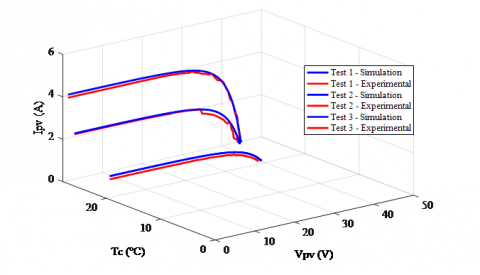## 3b.png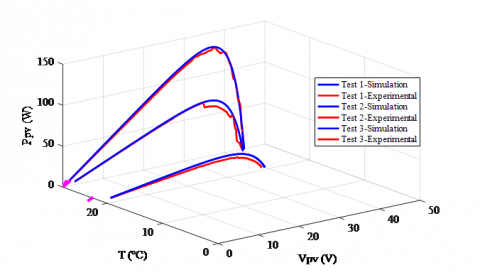Figure 3. Electrical characteristics

4.2 Wind turbine modeling

The wind power relationship is expressed in terms of wind speed as :

$P_{T b}=\frac{1}{2} \cdot C_{p} \cdot \rho \cdot \pi \cdot R_{T b}^{2} \cdot V_{\text {wind }}^{3}$          (12)

The maximum power is as follows:

$P_{T b-\max }=\frac{1}{2} \cdot C_{p_{-} \max } \cdot \rho \cdot \pi \cdot R_{T b}^{2} \cdot\left(\frac{\omega_{T b-o p t . R_{T b}}}{\lambda_{o p t}}\right)^{3}$                    (13)

The turbine torque is as :

$P_{T b-\max }=\frac{1}{2} \cdot C_{p_{-} \max } \cdot \rho \cdot \pi \cdot R_{T b}^{2} \cdot\left(\frac{\omega_{T b-o p t . R_{T b}}}{\lambda_{o p t}}\right)^{3}$         (14)

The permanent magnet synchronous machine model is :

${\left[\begin{array}{l}V_{d} \\ V_{q}\end{array}\right]=\left[\begin{array}{cc}R_{s} & -\omega \cdot L_{s} \\ \omega \cdot L_{s} & R_{s}\end{array}\right] \cdot\left[\begin{array}{c}I_{d} \\ I_{q}\end{array}\right]+L_{s} \frac{d}{d t}\left[\begin{array}{c}I_{d} \\ I_{q}\end{array}\right]+\left[\begin{array}{c}e_{d} \\ e_{q}\end{array}\right] }$

$T_{e m}=(3 / 2) P\left(L_{d}-L_{q}\right) I_{d} \cdot I_{q}+\Phi_{f} \cdot I_{q} \\ J \frac{d \omega}{d t}=T_{T b}-T_{e m}$              (15)

where, J is the total inertia in (kg.m²), TTb is the mechanical torque developed by the turbine in (N.m), Tem is the electromagnetic torque in (N.m) and P is the number of pairs of poles.

4.3 Battery modeling

Battery storage is very important to compensate the renewable energy (PV and wind) since weather conditions are variable and can be worse. The studied model is as shown in Figure 4 . Battery equations are as follows:

$V_{\text {batt }}=E_{\text {batt }} \pm R_{\text {batt }} \cdot I_{\text {batt }}$

$C_{\text {batt }}=C_{10} \times \frac{1.76 \times(1+0.005 \times \Delta T)}{1+0.67 \times\left(\frac{I_{\text {batt }}}{I_{10}}\right)} R_{\text {batt }} \cdot I_{\text {batt }}$

$\operatorname{SOC}(\%)=100 .\left(1-\frac{I_{\text {batt }} t}{C_{\text {batt }}}\right)$                 (16)

where, SOC is the battery state of charge.

## 4.png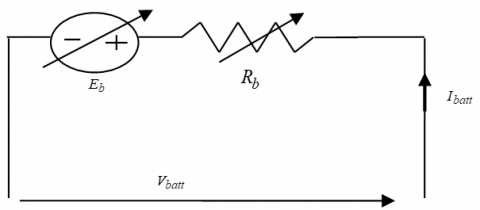Figure 4. Electrical model of lead acid battery

5. MPPT Algorithms

The strategy optimization regarding the PV system is made by three MPPT methods (Pertub& Observ (P&O), Incremental & conductance (INCcond) and fuzzy logic controller (FLC). And for the wind turbine, three MPPT methods have been investigated: (Optimal torque control (OTC), Gradient Method (GM) and Fuzzy logic controller (FLC).

5.1 Pertub and observe method (P&O)

One of the most common traditional approaches is the P&O algorithm. Its operation is determined by the tracking step size of the voltage reference . This method is very simple to be implemented and it does not require any knowledge of the photovoltaic parameters, but presents oscillations at steady state. The different steps are shown in Figure 5a.

When ΔPpv>0 and ΔVpv>0 thus ΔPpv∕ΔVpv>0, the duty cycle is incremented, D=DD.

When ΔPpv>0 and ΔVpv<0 thus ΔPpv∕ΔVpv<0, the duty cycle is decremented, D=D-ΔD.

When ΔPpv=0 and ΔVpv=0 thusΔPpv∕ΔVpv=0, we retain the duty cycle, ΔD=0, D=D.

5.2 Incremental conductance algorithm (IncCond)

This alternative technique for determining the MPP is based on the knowledge of the conductance of the solar generator change and of the effects on the position of the operating point when compared to a maximum power point . Its principle is to compare the conductance and the incremental conductance and to decide when to increase or to decrease the PV voltage to reach the MPP where the derivative of the power is equal to zero. The disadvantage of this method with fixed step is its oscillations. The different steps of the algorithm are displayed in Figure 5b.

When ΔIpv∕ΔVpv>-Ipv∕Vpv, the duty cycle is incremented D, D=D+ΔD.

When ΔIpv∕ΔVpv<-Ipv∕Vpv, the duty cycle is decremented D, D=D-ΔD.

When ΔIpv∕ΔVpv=-Ipv∕Vpv, the duty cycle is retained D, ΔD=0, D=D.

5.3 Fuzzy logic controller (FLC)

Fuzzification, inference engine, and defuzzification are the three steps that constitute the FLC. The error and change in error are the two inputs of the controller system. Its flowchart is depicted in Figure 5c for PV generators and Figure 5d for wind generators. It optimizes the magnitude of the increment to procure fast and fine tracking. The system based on MPPT fuzzy logic controller is composed of two inputs that are the error E(k) and the change in the error CE(k). For the PV generator, we have:

$\left\{ \begin{matrix} E(k)=\frac{{{P}_{pv}}(k+1)-{{P}_{pv}}(k)}{{{V}_{pv}}(k+1)-{{V}_{pv}}(k)} \\ CE(k)=E(k+1)-E(k) \\ \end{matrix} \right.$           (17)

For wind turbine generator the rules depend on the wind power variations and speed variations, which give a torque reference and a wind turbine reference speed:

\left\{\begin{aligned} \Delta \omega_{T b} &=\omega_{T b}(k)-\omega_{T b}(k-1) \\ \Delta P_{T b} &=P_{T b}(k)-P_{T b}(k-1) \\ \omega_{T b-r e f} &=\omega_{T b}(k-1)+\Delta \omega_{T b}(k) \end{aligned}\right.          (18)

where, PTb(k) andTb(k) are respectively the output turbine power and its rotational speed at sampled instants (k) and DTb,ref(k) the instant of reference speed.

5.4 Optimal torque control algorithm (OTC)

Optimal torque control will adapt the generator torque to its maximum at various wind speeds. However, the properties of the turbine have to be known. The OTC algorithm is depicted in Figure 5e. Optimal power, rotational speed and electromagnetic torque are given as:

\left\{\begin{aligned} P_{T b-o p t}=&(1 / 2) \cdot\left(C_{p-o p t} \cdot \rho \cdot \pi \cdot R_{T b}^{2}\right) \cdot V_{\text {wind }}^{3} \\ & \omega_{o p t}=V_{\text {wind }} \cdot \lambda_{o p t} / R_{T b} \\ T_{e m-o p t}=&(1 / 2) \cdot\left(C_{p} \cdot \rho \cdot \pi \cdot R_{T b}^{5} / \lambda 3\right) \cdot \omega_{T b-o p t}^{2} \end{aligned}\right.                 (19)

5.5 Gradient method algorithm (GM)

The search for the optimal point by this method does not need the knowledge of the parameters of the optimal tip speed ratio and maximal power coefficient. The reference turbine speed is increased to its maximum, resulting in maximum power. To act on the generator speed, we control the direction of variation of the ratio dPTb/dwTb (Figure 5f). When this ratio becomes equal to zero, the desired maximum power is reached. The algorithm requires the knowledge of the power and speed at all times. At the iteration k, the speed reference is increased or decreased by a fixed step ΔωTb.

$d P_{T b} / d \omega_{T b}=d P_{T b} / d t .\left(\omega_{T b} / d P_{T b}\right)^{-1}$         (20)

## 5a.png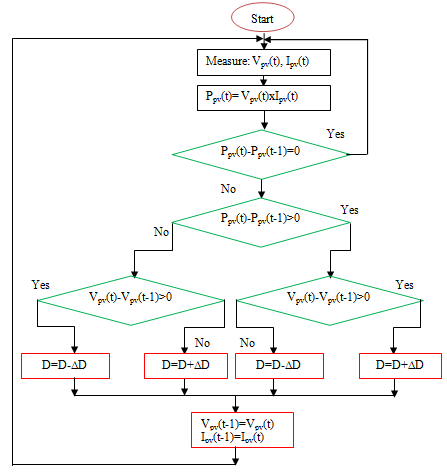(a) P&O

## 5b.png(b) IncCond

## 5c.png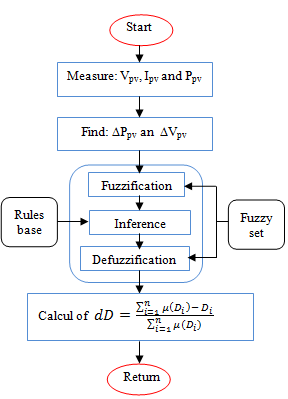(c) FLC for PV generator

## 5d.png(d) FLC for wind generator

## 5e.png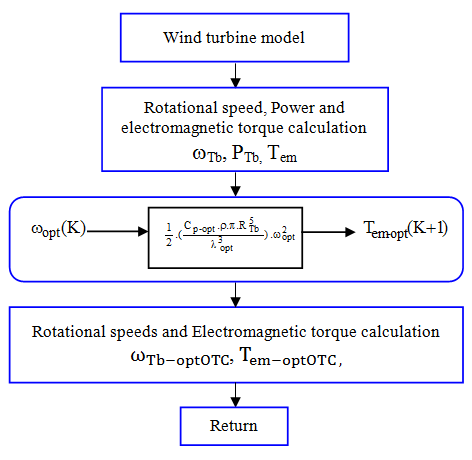(e) OTC

## 5f.png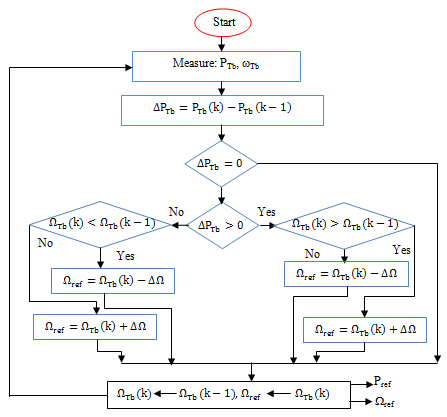(f) GM

Figure 5. Flowchart of P&O, IncCond and FLC in PV and wind generators, OTC and GM strategies

6. Simulation Study under MATLAB/SIMULINK

6.1 Results under step profiles of solar irradiance and wind speeds

6.1.1 PV system

P&O, FLC and IncCond methods were used in our study. A step profile was chosen (Figures 6 and 7).

## 6.png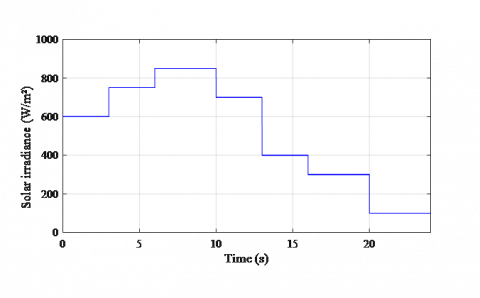Figure 6. Step profile of solar irradiance

## 7.png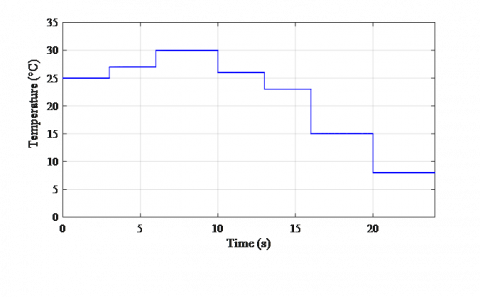Figure 7. Step profile of ambient temperature

The obtained powers are shown in Figure 8. The comparison of the different techniques in terms of efficiency, power, and response time are given in Figure 9. The FLC technique generates the best efficiency whatever the wind speed. The efficiency of each method is calculated as:

$\eta_{M P P T}(\%)=\frac{P_{o p t}}{P_{g}} x 100$           (21)

where, Popt and Pg are respectively the power with and without optimization.

## 8.pngFigure 8. Photovoltaic power under profile step

## 9a.png(a) Photovoltaic power

## 9b.png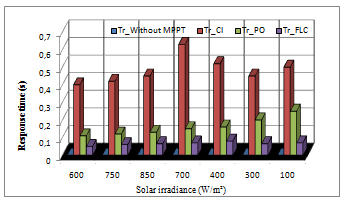(b) Response time for PV powers

## 9c.png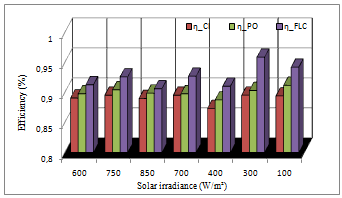(c) Efficiency

Figure 9. Photovoltaic MPPT comparisons

6.1.2 Wind turbine system

OTC, GM, and FLC methods are used in our study. A step profile is chosen (Figure 10).

## 10.png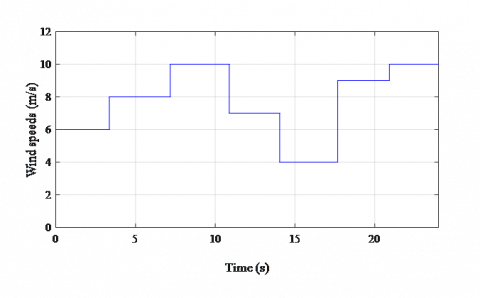Figure 10. Step wind speeds profile

The obtained powers are shown in Figure 11. A comparison of the different techniques in terms of efficiency, power, and response time is depicted in Figure 12. The FLC approach, when compared to the other methods, produces the highest power ratings for all the wind speeds ranging from 4 to 10 m/s.

## 11.png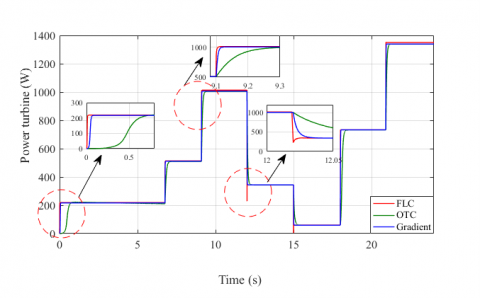Figure 11. Wind power under the three MPPT methods

## 12a.png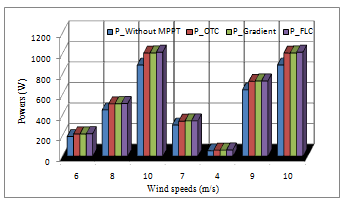(a) Wind turbine powers

## 12b.png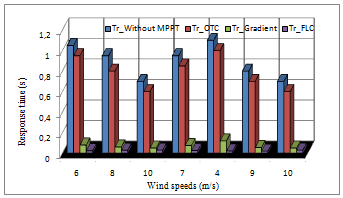(b) Response time

## 12c.png(c) Efficiency

Figure 12. Wind turbine MPPT comparisons

It is clear from the previous power results that the FLC approach gives the best efficiency for any wind speed. The suggested approaches have been successful in extracting the most PV and wind energy while keeping the best power coefficient, but the FLC method performed the best, and therefore it will be used in the following simulations.

6.2 Simulation results under variable days profile

Measurements of solar irradiance, temperature and wind speed were carried out in the laboratory by a measurement acquisition device The models are developed in Matlab/Simulink considering these measurements (Figure 13).

## 13a.png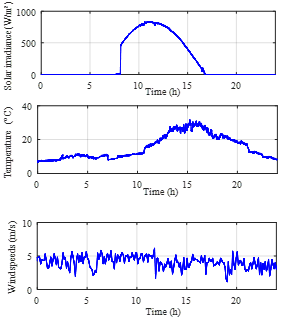(a) Day 1

## 13b.png(b) Day 2

## 13c.png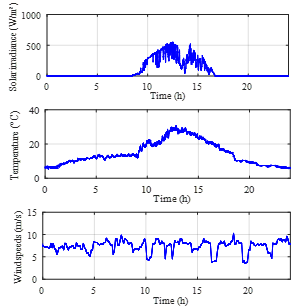(c) Day 3

## 13d.png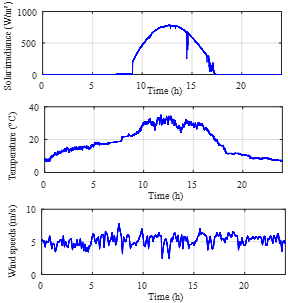(d) Day 4

Figure 13. Environmental conditions (solar irradiance, ambient temperature and wind speeds)

The obtained powers respectively for the PV and wind generators are given respectively in Figures 14-15.

## 14a.png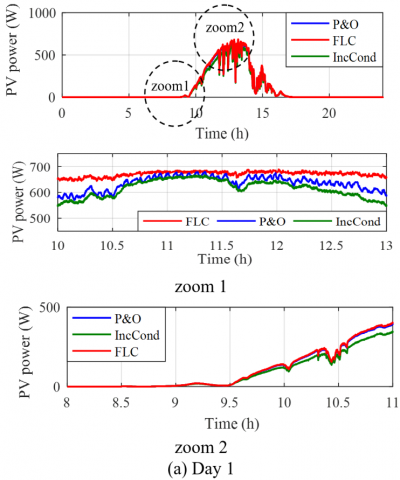## 14b.png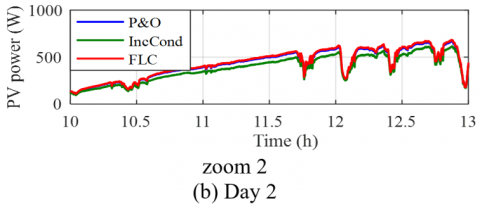## 14c.png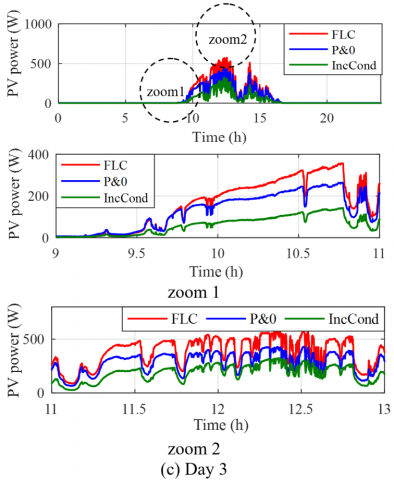## 14d.png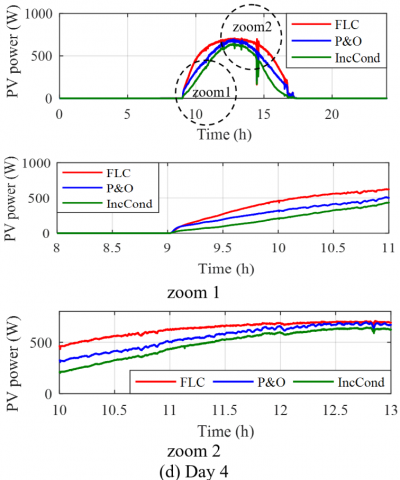Figure 14. Photovoltaic power during the four different days with their zooms

## 15a.png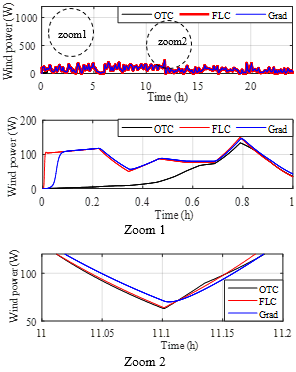(a) Day 1

## 15b.png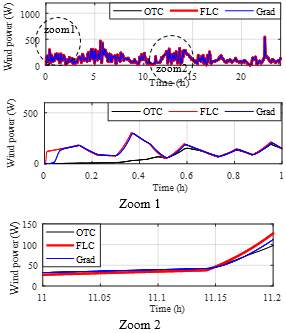(b) Day 2

## 15c.png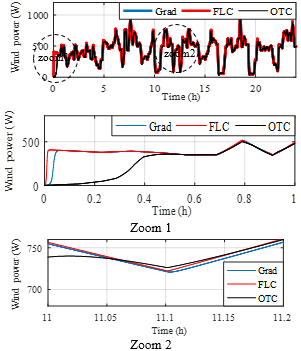(c) Day 3

## 15d.png(d) Day 4

Figure 15. Wind power during the four different days with their zooms

All of these data show that the FLC produces the best results in photovoltaic and wind generators in terms of power; thus, the FLC will be used for the hybrid system. During the four days, the hybrid power with FLC MPPT is provided (Figure 16).

## 16a.png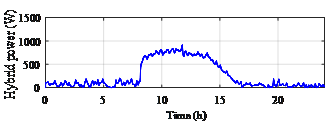(a) Day 1

## 16b.png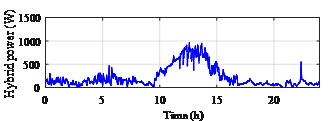(b) Day 2

## 16c.png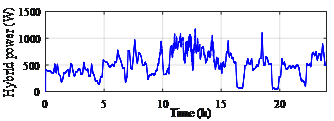(c) Day 3

## 16d.png(d) Day 4

Figure 16. Hybrid power

7. Power Control of the Proposed System

An application for water pumping is considered for the area of Bejaia, a coastal region in the east of Algeria. The daily average water use is 0.5 m3. The energy utilized per day is around 7445.51 Wh/day. The profile of the load is adjusted according to the following equation :

$P_{\text {Load }}=P_{P V}+P_{\text {wind }} \pm P_{\text {batt }}$      (22)

We have already made an application for a wind turbine system with batteries  and the proposed supervision has performed well and given good results. The same method was also applied to a PV system with batteries [23, 24] and to another application involving a PV system with batteries and fuel cells . The found results confirmed the feasibility of the proposed method. In this paper, an application to a hybrid water pumping system is sought. The proposed power management control (PMC) is shown in Figure 17.

## 17.png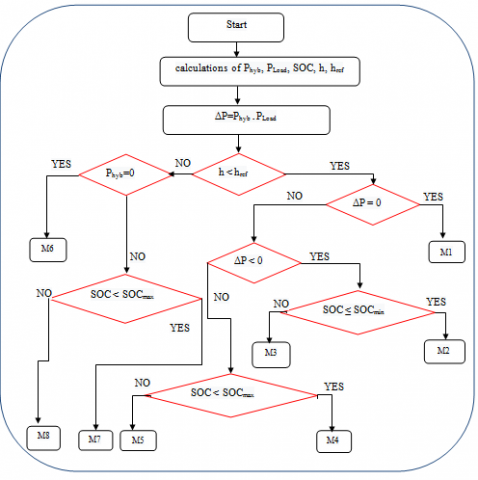Figure 17. Flowchart of the proposed PMC

There are eight operating modes suggested to satisfy the power.

The initial condition of the battery SOC is:

$\mathrm{SOC}_{\min }=30 \%\left\langle\mathrm{SOC}\left\langle\mathrm{SOC}{ }_{\max }=90 \%\right.\right.$        (23)

Mode 1 (M1): Phyb=Pload and the height is less than the reference height, which corresponds to the maximum volume of water to be pumped. The motor pump unit is driven by photovoltaic and wind powers.

Mode 2 (M2): Phyb<Pload, SOC≤30%, the height is lower than the reference height, which corresponds to the maximum volume of water to be pumped. The batteries are then disconnected, and as the requirement of the load has not been met, the latter is immediately unloaded.

Mode 3 (M3): This situation occurs when the height is less than the reference height, which corresponds to the maximum volume of water to be pumped, and Phyb<Pload, SOC>30%. The batteries will compensate for the shortage of power.

Mode 4 (M4): It corresponds to a height lower than the reference height which corresponds to the maximum volume of water, and more Phyb>Pload and SOC≤90%. The load will be driven by the electricity generated by the two generators. The remaining energy is used to recharge the batteries.

Mode 5 (M5): The height is lower than the reference height which corresponds to the maximum volume of water (Phyb>Pload and SOC>90%). The load will be driven by the power generated by the two generators and the surplus will be dissipated in a dump load.

Mode 6 (M6): It occurs when the head is greater than or equal to the reference head, which corresponds to the maximum amount of water that can be pumped. There is no need to fill the tank in this case. Also Phyb=0, the load as well as the batteries will be disconnected.

Mode 7 (M7): The height is greater than or equal to the reference height which corresponds to the maximum volume of water. There is no need to fill the water tank. Moreover Phyb≠0 and SOC<90%. The power generated by the photovoltaic and wind generators will be used to charge the batteries.

Mode 8 (M8): The height is greater than or equal to the reference height which corresponds to the maximum volume of water. There is no need to fill the water tank. Moreover Phyb≠0 and SOC>90%. In this case the batteries will be disconnected.

The simulation program is run under Matlab/Simulink environment. The chosen height is variable (Figure 18) and the power of the pump is of about 900 W (Figure 2).

## 18.pngFigure 18. Chosen reference heights

A water pumping was realized during 8.28 hours maximum for each day. The pump power is given in Figure 19. The state of charge and battery voltage are respectively shown (Figure 20 and 21). It can be observed that the batteries (in red color) have not been solicited much whatever the period of the year. Indeed, the solar irradiance and the wind speeds in this Mediterranean region are very complementary especially in the summer (strong solar irradiance and low wind speeds) and in the winter (high wind speeds and average solar irradiance).

## 19.png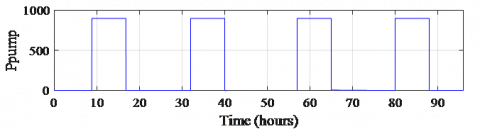Figure 19. Power of the water pumping

## 20.png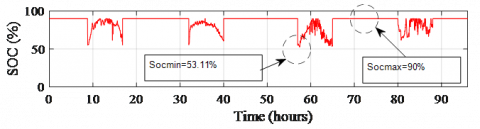Figure 20. Battery state of charge

## 21.png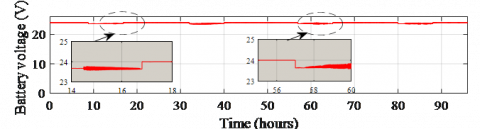Figure 21. Battery voltage

The different powers of each day are as follows (Figure 22).

## 22a.png(a) Summer day

## 22b.png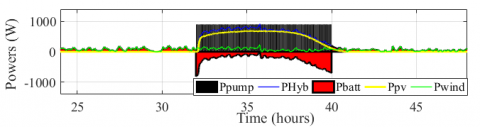(b) Autumn day

## 22c.png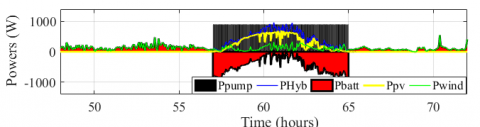(c) Winter day

## 22d.png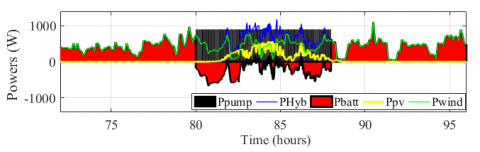(d) Spring day

Figure 22. Obtained different powers for each day

It can be noted that the batteries have not been solicited much whatever the period of the year. The SOC values vary between 53.1% and 90%. The batteries were protected due to the proposed power control and the pump was supplied during 8.28 hours per day. The different powers have been well managed and the pump has been supplied with less stress on batteries.

8. Conclusions

Modeling, design and optimization of a hybrid photovoltaic/wind turbine/batteries system are described and evaluated in this work. This system allows us to satisfy the pump load and to protect the batteries used. This has been achieved mainly through the control, the correct and precise sizing provided by the proposed sizing method and of course through the FLC optimization strategy. These three parameters are important factors for the feasibility of this system. Matlab/Simulink simulation results verify the effectiveness and feasibility of the proposed control strategy. The main novelties and goals of this study can be affirmed as a significant power gain in PV and wind turbine powers, so less stress on the batteries of the system. In fact, this is on the one part to the proposed precise sizing method and accurate mathematical models and on the other part to the fuzzy logic control algorithm and the used power control method. In our future research, we intend to implement the proposed system with power management system and FLC optimization.

Nomenclature
 Cbatt battery capacity, Ah D duty cycle daut days of autonomy, days Eirr solar irradiance on a tilted plane, kWh/m2 ELoad,ave. annual average monthly energy value of the load, kWh/m2 Epv,ave annual average monthly energy value of PV generator, kWh/m2 Ewind,ave annual average monthly energy value of wind generator, kWh/m2 f fraction of power Impp maximum current at PPM, A Isc short-circuit current, A J total inertia, kg.m² Ls stator inductance, H m month of the year NBatt number of batteries Nm days number (31 days), Npv number of photovoltaic panels Nwind number of wind turbines P number of pole pairs Pbatt battery power, W Pi(i=1,4) constant parameters PDP depth of discharge Phyb hybrid power (PV and wind), W Pload load power, W PN rated power, W PPV photovoltaic power, W PTb turbine power, W Popt optimized power, W Rbatt internal resistance, Ω Rs resistance of the stator winding, Ω RTb turbine radius, m Spv photovoltaic area, m² Swind wind turbine area, m² Tem electromagnetic torque, N.m TTb mechanical turbine torque, N.m Vbatt battery voltage, V Vd direct axis stator voltage, V Vq quadrature axis stator voltage, V Vmpp maximum voltage at PPM, V Voc open-circuit voltage, V Vwind wind speeds, m/s Greek symbols αsc short-current temperature coefficient, A/K βoc voltage temperature coefficient, V/K $\Delta t$ period of time, hours $\eta_{b a t t}$ battery efficiency, % ηpv PV panel efficiency, % ηMPPT-PV PV MPPT efficiency, % ηMPPT-Tb wind turbine MPPT efficiency, % $\omega$ rotor electrical pulse, rad/s Subscripts FLC Fuzzy logic controller INCcond Incremental conductance GM Gradient method OTC Optimal torque control MPPT Maximum power point tracking NOCT Nominal operating cell temperature PMSG Permanent magnet synchronous generator P&O Perturb and Observe SOC State of charge
Appendix

Rated parameters of the used PV panel of 175 Wp:

PPV=175 Wp, Impp=4.95 A, Vmpp=35.4 V, Isc=5.40A, Voc=44.4 V, $\eta_{\mathrm{pv}}$=13.5%, αsc=+0.053 A/°C, βoc=-156.00V/°C, NOCT=47.5°C.

References

 Rekioua, D., Matagne, E., (2012). Optimization of photovoltaic power systems: Modelization, Simulation and Control. Series: Green Energy and Technology. Edition Springer. https://doi.org/10.1007/978-1-4471-2403-0

 Amrouche, S.O., Rekioua, D., Rekioua, T., Bacha, S. (2016). Overview of energy storage in renewable energy systems. International Journal of Hydrogen Energy, 41(45): 20914-20927. http://dx.doi.org/10.1109/IRSEC.2015.7454988

 Achour, A., Rekioua, D., Mohammedi, A., Mokrani, Z., Rekioua, T., Bacha, S. (2016). Application of direct torque control to a photovoltaic pumping system with sliding-mode control optimization. Electric Power Components and Systems, 44(2):172-184. http://dx.doi.org/10.1080/15325008.2015.1102182

 Zainuri, M.A.A.M., Radzi, M.A.M., Che Soh, A., Rahim, N.A. (2014). Development of adaptive perturb and observe-fuzzy control maximum power point tracking for photovoltaic boost dc-dc converter. IET Renewable Power Generation, 8(2): 183-194. http://dx.doi.org/10.1049/iet-rpg.2012.0362

 Rekioua, D., Rekioua, T., Soufi, Y. (2015). Control of a grid connected photovoltaic system. In 2015 International Conference on Renewable Energy Research and Applications (ICRERA), pp. 1382-1387. http://dx.doi.org/10.1109/ICRERA.2015.7418634

 Radhia, G., Mouna, B.H., Lassaad, S., Barambones, O. (2013). MPPT controller for a photovoltaic power system based on increment conductance approach. In 2013 International Conference on Renewable Energy Research and Applications (ICRERA), pp. 73-78. http://dx.doi.org/10.1109/ICRERA.2013.6749729

 Khadidja, S., Mountassar, M., M'hamed, B. (2017). Comparative study of incremental conductance and perturb & observe MPPT methods for photovoltaic system. In 2017 International Conference on Green Energy Conversion Systems (GECS), pp. 1-6. http://dx.doi.org/10.1109/GECS.2017.8066230

 Xing, C., Xi, X., He, X., Liu, M. (2020). Research on the MPPT control simulation of wind and photovoltaic complementary power generation system. In 2020 IEEE Sustainable Power and Energy Conference (iSPEC), pp. 1058-1063. http://dx.doi.org/10.1109/iSPEC50848.2020.9350965

 Kordestani, M., Mirzaee, A., Safavi, A.A., Saif, M. (2018). Maximum power point tracker (MPPT) for photovoltaic power systems-A systematic literature review. In 2018 European Control Conference (ECC), pp. 40-45. http://dx.doi.org/10.23919/ECC.2018.8550117

 Salas, V., Olias, E., Barrado, A., Lazaro, A. (2006). Review of the maximum power point tracking algorithms for stand-alone photovoltaic systems. Solar Energy Materials and Solar Cells, 90(11): 1555-1578. http://dx.doi.org/10.1016/j.solmat.2005.10.023

 Belaid, S., Rekioua, D., Oubelaid, A., Ziane, D., Rekioua, T. (2022). Proposed Hybrid Power Optimization for Wind Turbine/Battery System, Periodica polytechnica Electrical engineering and computer science, 66(1): 60-71. http://dx.doi.org/10.3311/PPee.18758

 Cui, Z., Song, L., Li, S. (2017). Maximum power point tracking strategy for a new wind power system and its design details. IEEE Transactions on Energy Conversion, 32(3): 1063-1071. http://dx.doi.org/10.1109/TEC.2017.2694008

 Koutroulis, E., Kalaitzakis, K. (2006). Design of a maximum power tracking system for wind-energy-conversion applications. IEEE Transactions on Industrial Electronics, 53(2): 486-494. http://dx.doi.org/10.1109/TIE.2006.870658.

 Taraft, S., Rekioua, D., Aouzellag, D., Bacha, S. (2015). A proposed strategy for power optimization of a wind energy conversion system connected to the grid. Energy Conversion and Management, 101: 489-502. http://dx.doi.org/10.1016/j.enconman.2015.05.047

 Idjdarene, K., Rekioua, D., Rekioua, T., Tounzi, A. (2011). Wind energy conversion system associated to a flywheel energy storage system. Analog Integrated Circuits and Signal Processing, 69(1): 67-73. http://dx.doi.org/10.1007/s10470-011-9629-2

 Aissou, R., Rekioua, T., Rekioua, D., Tounzi, A. (2016). Robust nonlinear predictive control of permanent magnet synchronous generator turbine using Dspace hardware. International Journal of Hydrogen Energy, 41(45): 21047-21056. http://dx.doi.org/10.1016/j.ijhydene.2016.06.109

 Aberbour, A., Idjdarene, K., Tounzi, A. (2020). Performance analysis of a self-excited induction generator mathematical dynamic model with magnetic saturation, cross saturation effect and iron losses. Mathematical Modelling of Engineering Problems, 7(4): 527-538. http://dx.doi.org/10.18280/mmep.070404

 Mebarki, N., Rekioua, T., Mokrani, Z., Rekioua, D. (2015). Supervisor control for stand-alone photovoltaic/hydrogen/battery bank system to supply energy to an electric vehicle. International Journal of Hydrogen Energy, 40(39): 13777-13788. http://dx.doi.org/10.1016/j.ijhydene.2015.03.024

 Rekioua, D., Rekioua, T. (2009). DSP-controlled direct torque control of induction machines based on modulated hysteresis control. In 2009 International Conference on Microelectronics-ICM, pp. 378-381. http://dx.doi.org/10.1109/ICM.2009.5418603

 Rekioua D., Bensmail S., Bettar N.(2014).Development of hybrid photovoltaic-fuel cell system for stand-alone application. International Journal of Hydrogen Energy, 39 (3): 1604-1611.

http://dx.doi.org/10.1016/j.ijhydene.2013.03.040

 Belaid, S., Rekioua, D., Oubelaid, A., Ziane, D., Rekioua, T. (2022). A power management control and optimization of a wind turbine with battery storage system. Journal of Energy Storage, 45: 103613. https://doi.org/10.1016/j.est.2021.103613

 Mohammedi, A., Rekioua, D., Rekioua, T., Bacha, S. (2016). Valve Regulated Lead Acid battery behavior in a renewable energy system under an ideal Mediterranean climate. International Journal of Hydrogen Energy, 41(45): 20928-20938. http://dx.doi.org/10.1016/j.ijhydene.2016.05.087

 Zaouche, F., Rekioua, D., Mokrani, Z. (2017). Power flow management for stand alone PV system with batteries under two scenarios. In 2017 International Renewable and Sustainable Energy Conference (IRSEC), pp. 1-6. http://dx.doi.org/10.1109/IRSEC.2017.8477328

 Rekioua, D. (2018). Energy Management for PV Installations. Advances in Renewable Energies and Power Technologies, 1: 349-369. https://doi.org/10.1016/B978-0-12-812959-3.00011-3

 Hassani, H., Zaouche, F., Rekioua, D., Belaid, S., Rekioua, T., Bacha, S. (2020). Feasibility of a standalone photovoltaic/battery system with hydrogen production. Journal of Energy Storage, 31: 101644. http://dx.doi.org/10.1016/j.est.2020.101644